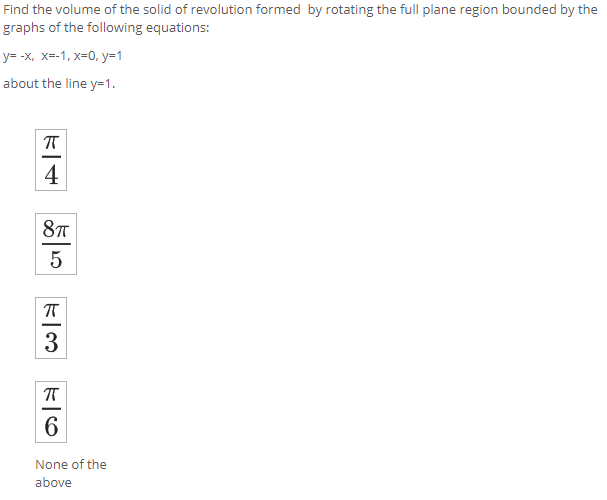### Create an Account

Home / Questions / Find the volume of the solid of revolution formed by rotating the full plane region bounde...

# Find the volume of the solid of revolution formed by rotating the full plane region bounded by the graphs of the following equations: y=-X, X=-1, x=0, y=1 about the line y=1. T 4 87 5 T 3 TT 6 None of

Find the volume of the solid of revolution formed by rotating the full plane region bounded by the graphs of the following equations: y=-X, X=-1, x=0, y=1 about the line y=1. T 4 87 5 T 3 TT 6 None of the aboveJun 03 2021 View more View LessSubscribe To Get Solution